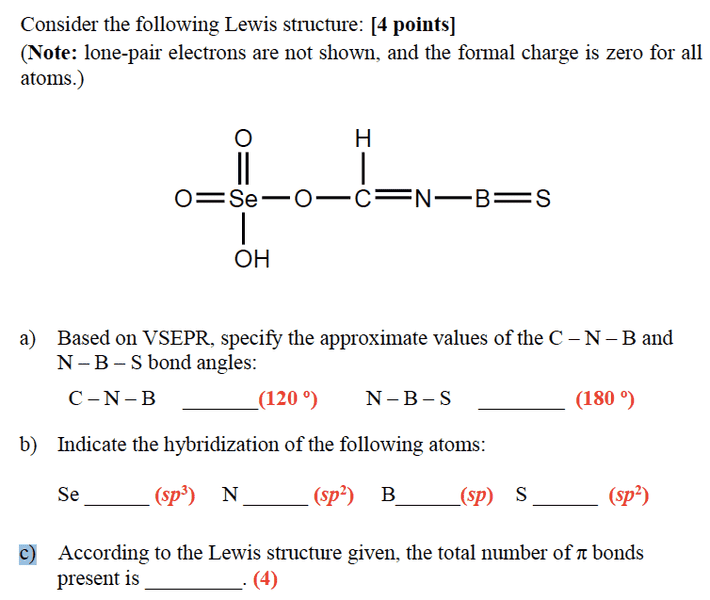# Number of Pi Bonds: MO Model

• yecko

Gold Member

## Homework Statementhttp://i.imgur.com/HunOeH0.png

MO model

## The Attempt at a Solution

as far as i know, number of pi bond is equal to 3 - the "power" of p (the hybridized bond formed)
the answer "4" is only calculated from the 4 elements' bond in part b, so why the central O and C atom is not considered with calculation of pi bond? Thanks!

•